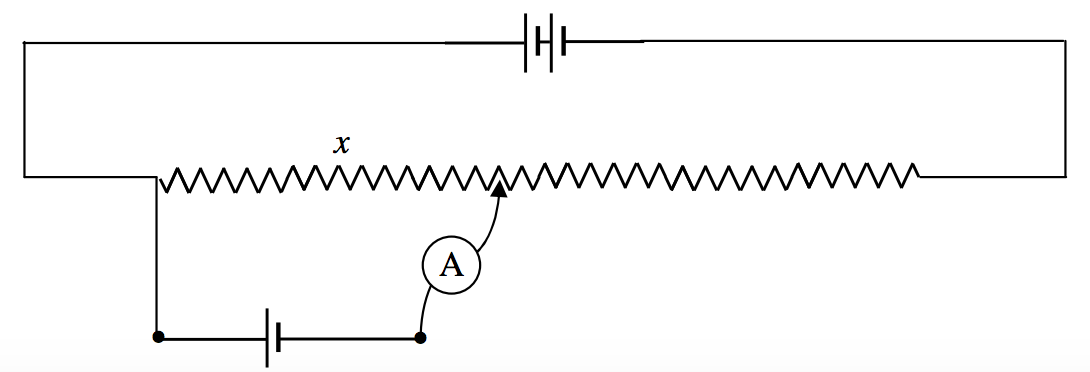Skip to main content
$$\require{cancel}$$

# 4.9: Potential Divider

•• Contributed by Jeremy Tatum
• Emeritus Professor (Physics & Astronomy) at University of Victoria

The circuit illustrated in figure IV.6 is a potential divider. It may be used to supply a variable voltage to an external circuit. It is then called a rheostat. Or it may be used to compare potential differences, in which case it is called a potentiometer. (In practice many people refer to such a device as a “pot”, regardless of the use to which it is put.)$$\text{FIGURE IV.6}$$

For example, in figure IV.7, a balance point (no current in the ammeter, A) is found when the potential drop down the length x of the resistance wire is equal to the EMF of the small cell. (Note that, since no current is being taken from the small cell, the potential difference across its poles is indeed the EMF.) One could compare the EMFs of two cells in this manner, one of which might be a “standard cell” whose EMF is known.$$\text{FIGURE IV.7}$$ :The potentiometer is being used as a rheostat to supply a variable voltage to an external circuit.

In figure IV.8, a current is flowing through a resistor (which is assumed to be in part of some external circuit, not drawn), and, assuming that the potential gradient down the potentiometer has been calibrated with a standard cell, the potentiometer is being used to measure the potential difference across the resistor. That is, the potentiometer is being used as a voltmeter.$$\text{FIGURE IV.8}$$ : The potentiometer is being used as a voltmeter